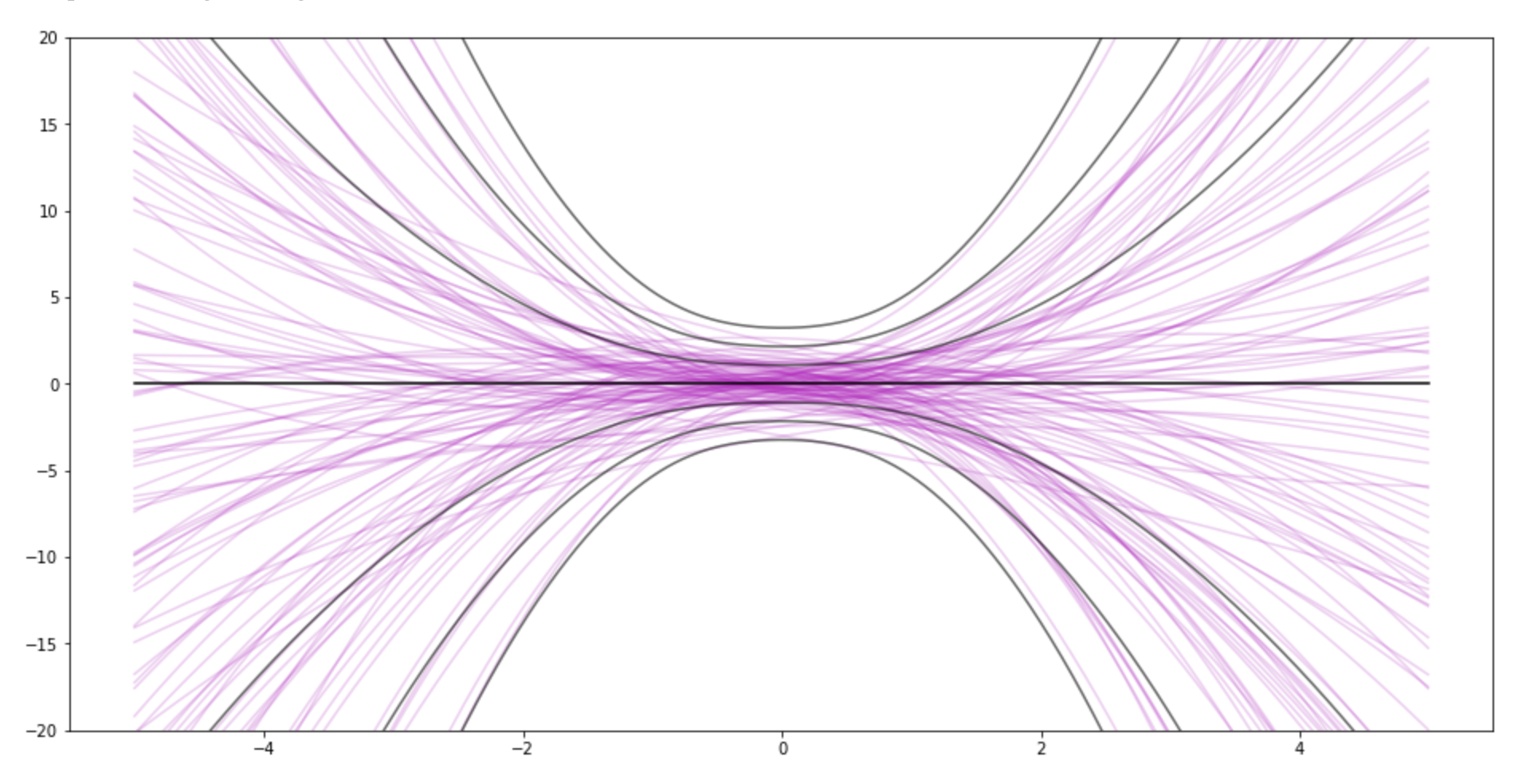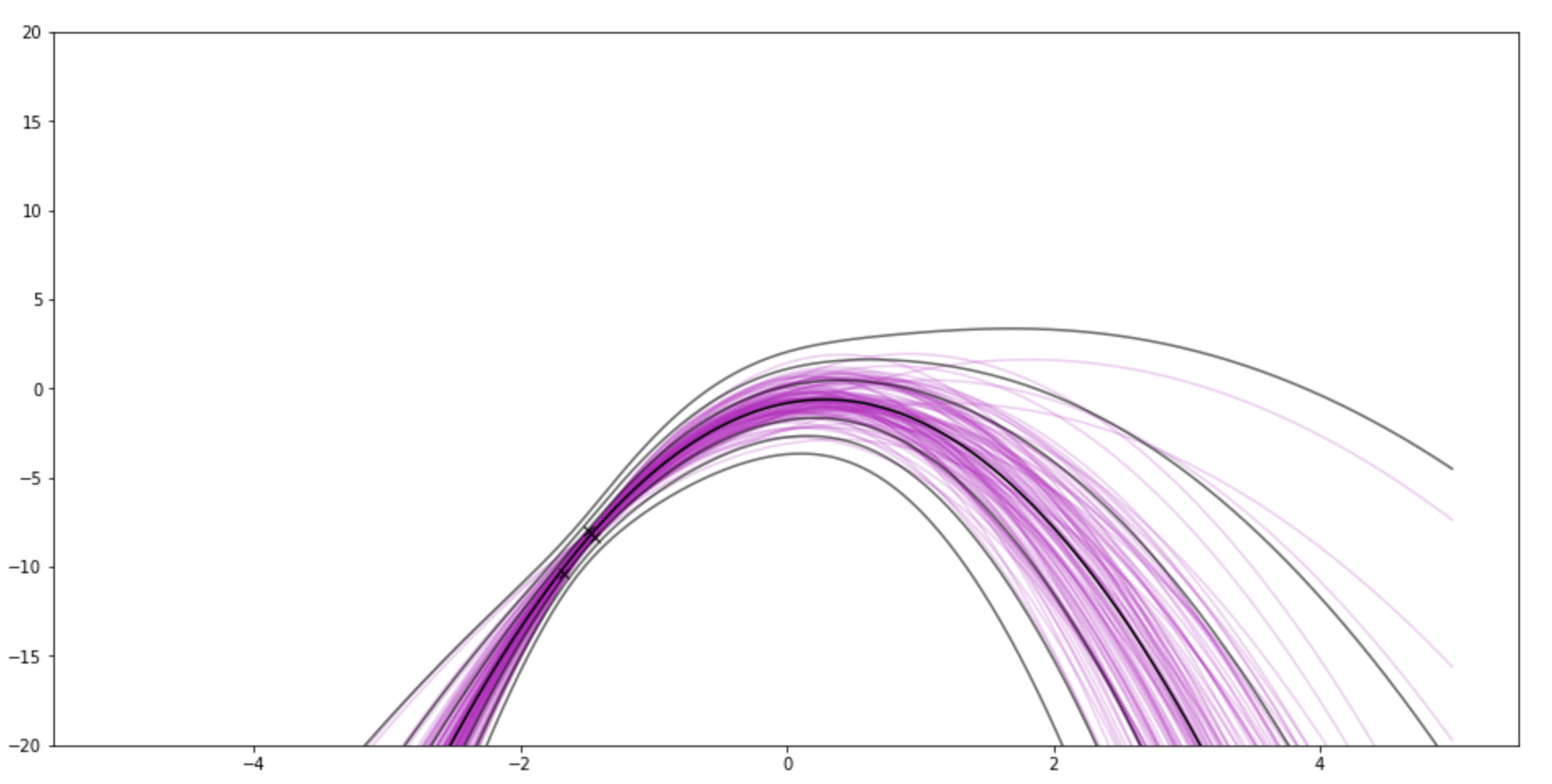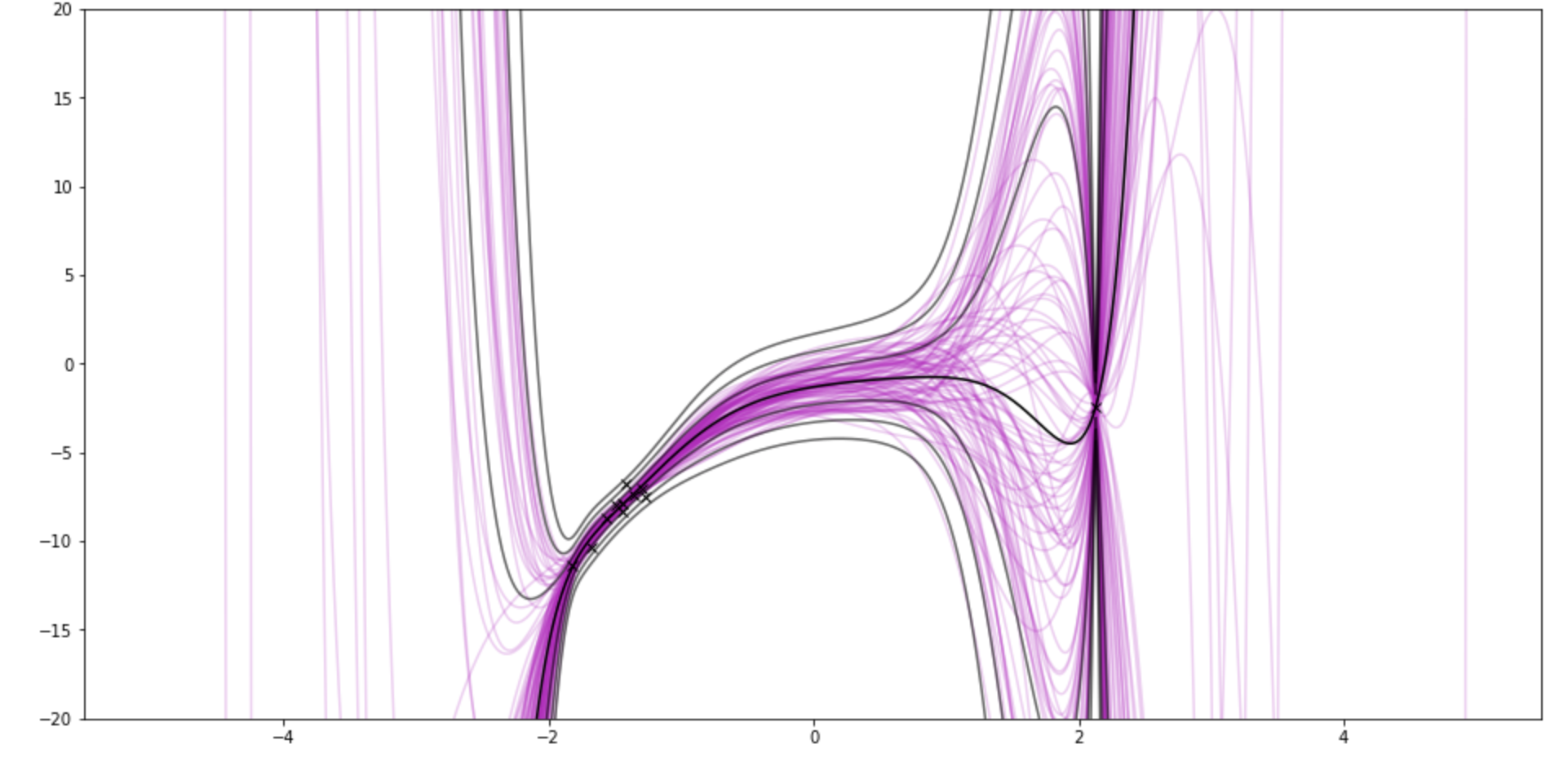Skip to content
{{ message }}

Instantly share code, notes, and snippets.

# jessstringham/0.notes.md

Last active Nov 17, 2017
Bayesian linear regression with polynomial basis functions

Srry, this is a bit of an awkward format. I might move this and a few other demos to a repo.

This is code one could drop into Jupyter  notebook cells and draw some wicked-looking graphs. I wrote it to understand Bayesian linear regression (for this classMLPR, specifically this and this). No promises that I did it perfectly.

The graphs show samples from the posterior weights in pink. The black lines kinda show the posterior distribution P(y|data) by drawing lines for standard deviations away from the mean. So it goes through the points on the x-axis, asks P(y|data) for the value and uncertainty

It uses polynomial basis functions, because they are sqiggly and cool-looking.

• model_params=3 matches the underlying function (and the right noise variance sigma_y is already set)
• If you set "sample size" to 0, you can see the prior. Then try messing with sigma_w. This demo uses the same sigma_w for all weights. You can also see the impact of the prior after data.
• For fun, I sample from two regions of the underlying function. So try going from sample_size 10 to 11.
• If you set the "model–params" (number of coefficients) to 10 and sample size to 11, it pinches around the brand new point.

 with matplotlib, numpy, scipy

 import matplotlib.pyplot as plt import numpy as np from ipywidgets import interact, interact_manual from scipy.linalg import cholesky from scipy.stats import multivariate_normal # weights for the underlying function real_w = np.vstack((0.5, 3, -2)) def generate_noisy_samples(sample_size, actual_sigma_y, real_w): # sample sample_size points between -2, 2 #X_in = np.random.rand(sample_size, 1) * 4 - 2 # sample approx sample_size points near -2 and 2 X_in = np.vstack(( np.random.rand(int(sample_size / 2), 1) - 2, np.random.rand(int(sample_size / 2), 1) + 2 )) f = np.hstack(X_in**i for i in range(len(real_w))) @ real_w noise = actual_sigma_y * np.random.randn(X_in.shape, 1) y = f + noise assert y.shape == (sample_size, 1) return X_in, y all_X_in, all_y = generate_noisy_samples( sample_size=20, actual_sigma_y=.4, real_w=real_w, )
 N = 100 # number of lines grid_size = 0.01 x_grid = np.arange(-5, 5, grid_size) def plot_example(model_params, sample_size, sigma_w): # For fun graphics, we use the first `sample_size` points. X_in = all_X_in[:sample_size] y = all_y[:sample_size] # Set our guesses of sigma #sigma_w = 1 # weights sigma_y = .4 # noisy observations # Set up the prior w_0 = np.tile(0, (model_params, 1)) V_0 = sigma_w**2 * np.identity(model_params) # Compute phi for our X_in Phi_X_in = np.hstack(X_in**i for i in range(model_params)) # Now compute the posterior, just reading off the formula V0_inv = np.linalg.inv(V_0) V_n = sigma_y**2 * np.linalg.inv(sigma_y**2 * V0_inv + (Phi_X_in.T @ Phi_X_in)) w_n = V_n @ V0_inv @ w_0 + 1 / (sigma_y**2) * V_n @ Phi_X_in.T @ y assert w_n.shape == (model_params, 1) # Now let's draw! # Now we're ready to start plotting. # This is the X for the entire grid X = np.vstack(x_grid**i for i in range(model_params)).T plt.clf() plt.figure(figsize=(16, 8)) # here are some posterior lines w = np.random.multivariate_normal(mean=w_n.flatten(), cov=V_n, size=N) plt.plot(x_grid, X @ w.T, '-m', alpha=.2) # here's the mean plt.plot(x_grid, X @ w_n, '-w') # How curvey can this be? var_post = np.sum(np.dot(X, V_n) * X, 1)[:, None] + sigma_y**2 for k in np.arange(1, 4): plt.plot(x_grid, X @ w_n + k * np.sqrt(var_post), '-', color='0.2') plt.plot(x_grid, X @ w_n - k * np.sqrt(var_post), '-', color='0.2') # here is the prior #plt.plot(x_grid, X @ w_0, '-g') # here's f #plt.plot(x_grid, np.vstack([x_grid**i for i in range(len(real_w))]).T @ real_w, '-b') # here is samples plt.plot(X_in, y, 'xk') # here are the points used in the posterior plt.ylim([-20, 20]) plt.show() interact( plot_example, model_params=(1, 10), sample_size=(0, 20), sigma_w=(0.1, 3), continuous_update=False, )

###jessstringham commented Nov 17, 2017

 This is the prior for 3 weights (quadratic)After 3 observations. There's still a little lump around 0.If I use 9-degree polynomial, and show 11 samples (10 from one side, 1 from another side)to join this conversation on GitHub. Already have an account? Sign in to comment